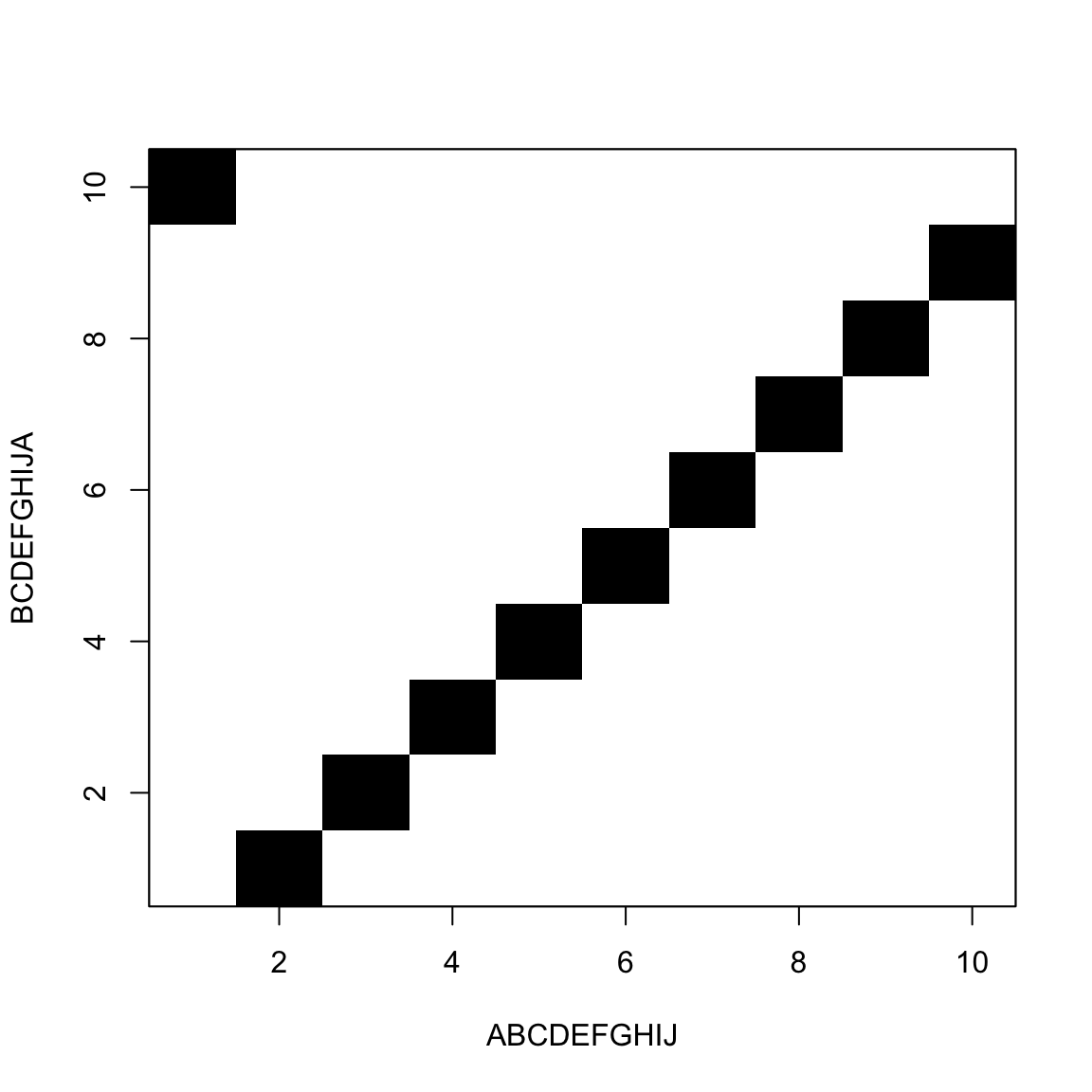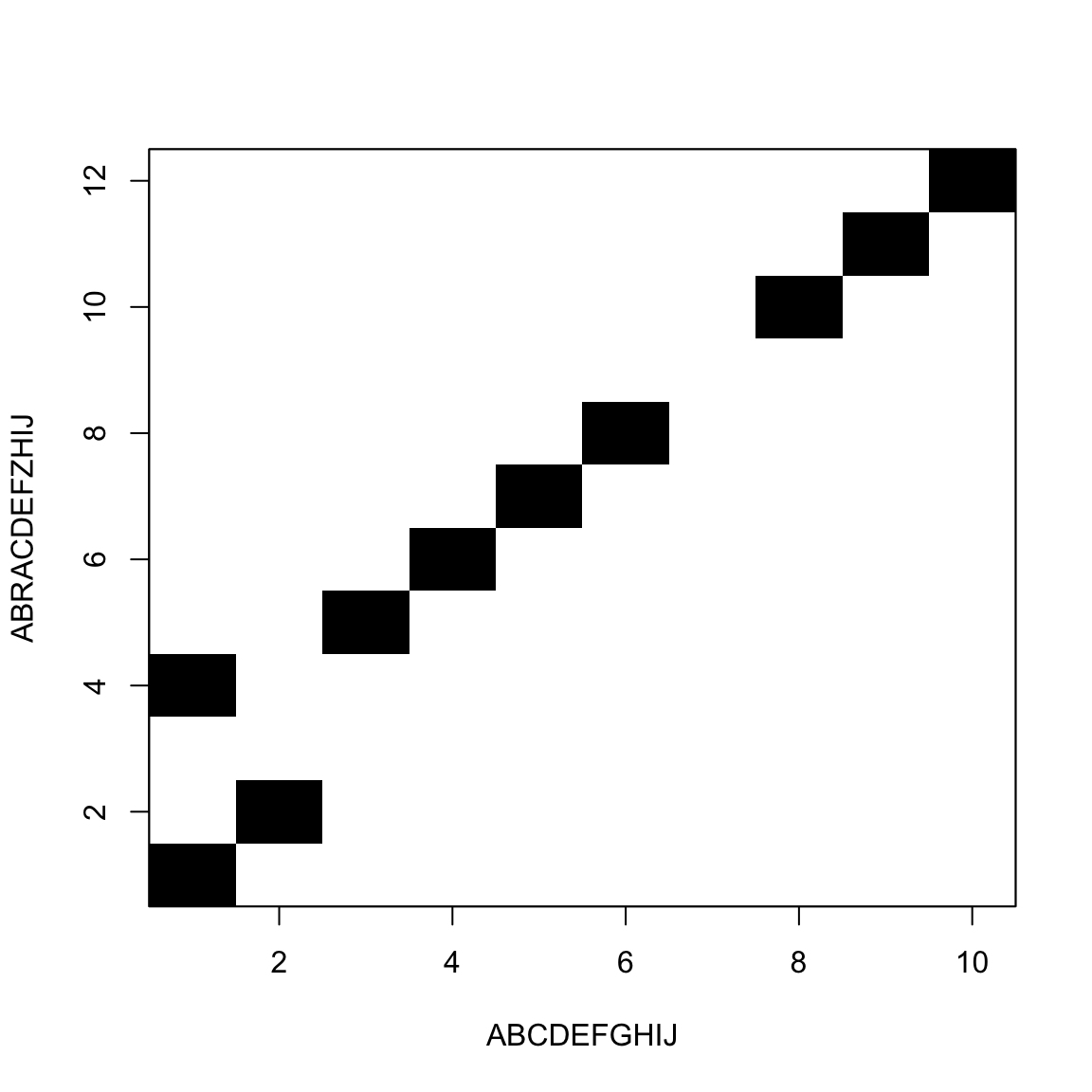# Bioinformatics

## How does spell check work?

When you write a text in any good text editor
(or even in Microsoft Word®),
some words are automatically underlined with red

Sometimes the editor can suggest the correct word

How does the computer do that?

## How do we find the suggestion?

One option is to define a rule, like a program, that will tell if a word is correct or not $isCorrect: Words ↦ \{True, False\}$ That is hard, in general

## There is an easier way

Other option is to have a dictionary or database of know correct words, and a way to find the nearest word $Similar: (Words, Database) ↦ \{Words\in Database\}$

## What do we mean by similar?

When are two sequences similar?

## Calculating a distance between strings

First idea:

• We count the number of mismatches

• Both strings must have the same length

  ASELLKYLTT
ASELLKALTT

Here distance(ASELLKYLTT,ASELLKALTT) is 1

## Hamming distance examples

How many substitutions we need to go from one sequence to the other

• CAT and CAT have a Hamming distance of 0
• CAT and BAT have a Hamming distance of 1
• CAT and BAG have a Hamming distance of 2

## Let’s make a Hamming distance calculator in Excel or Google Sheets

Let’s pause a minute and do this exercise

## What if sequences have different length?

In that case we need to insert “gaps”

MOUSE
GROUSE

## There are many ways to insert gaps

The distance depends on the gaps positions

-MOUSE
GROUSE

Hamming Distance=2

MOUSE--
-GROUSE

Hamming Distance=7

## So, which one is the distance?

In geometry we say that “the distance between a point X and a line L is the shortest length from X to any point in L”

## Same idea

If there many “candidate distances”, we choose the smallest one

This is an important idea

## Now we also count gaps

Hamming distance counts substitutions between sequences

Now we counts substitutions, insertions and deletions

## Hamming versus Levenstein

Hamming distance counts substitutions

ABCDEFGHIJ
BCDEFGHIJA

Hamming distance=10

Levenstein counts substitutions, insertions and deletions

ABCDEFGHIJK-
-BCDEFGHIJKA

Levenstein distance=2

## What is the best way to insert gaps?

If the sequence has m letters, there are 2m+1 ways to insert a single gap

CAT
CAT-
CA-T
CA-T-
C-AT
C-AT-
C-A-T
C-A-T-
-CAT
-CAT-
-CA-T
-CA-T-
-C-AT
-C-AT-
-C-A-T
-C-A-T-

## And that is not even counting larger gaps

We could also do

C--A-----T

or

----CA-T--

or

-C--A--T--

## There is a better way to find the best

We will see more details later

The idea is to draw a rectangle

• One sequence in the rows
• Another sequence in the columns
• Mark the cells where row letter and column letter are equal

## It looks like thisThe goal is to move from one corner to the other

Jumping black to black is free

Horizontal and vertical moves are gaps

## Exercise

Prepare a DotPlot in Excel or Google Sheets

## We move from one corner to the other## White blocks add to the distance## Summary

• Hamming distance
• Levenstein distance
• Dot plot